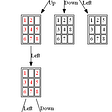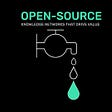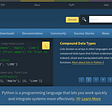# 1. Data Types

Python is an extremely popular language which is primarily used in Data Science, Web Development and Bioscience. The syntax is known to be easy to read and learn and has a lot of similarities to the English Language.

• New lines are used to indicate the end of a conditional statement, loop or function
• Indentation is used to indicate scope (e.g. a piece of code will only run within a function)
• Variables are storage containers for different types of data
`example_variable = 12 # Integer -> intexample_variable = "Hello World" # String -> strexample_variable = 12.4 # Float -> floatexample_variable = complex(2, -3) # Complex -> complexexample_variable = [1, 2, 3] # List -> listexample_variable = (1, 2, 3) # Tuple -> tupleexample_variable = range(5) # Range -> rangeexample_variable = {"element1" : 1} # Dictionary -> dictexample_variable = True # Boolean -> bool`

# 2. Printing

Prints a message onto the screen

`print("Hello World")`

# 3. Strings

A sequence of characters

## Creating

`example_string = "Hello World"print(example_string) # OUTPUT: Hello World`

## Concatenation

`first_string = "Hello "second_string = "World"third_string = first_string + second_stringprint(third_string) # OUTPUT: Hello World`

## Slicing

`example_string = "Hello World"print(example_string[0:5]) # OUTPUT: Hello# Slice using [firstIndex : secondIndex + 1]# ∴ [0:5] is the first 5 characters (0th to 4th index)`

## Substitution

`example_string = "Hello World"print("My string is", example_string)# OUTPUT: My string is Hello World`

## Special Methods

`example_string = "Hello World"print(example_string.upper())# OUTPUT: HELLO WORLDprint(example_string.lower())# OUTPUT: hello worldprint(type(example_string))# OUTPUT: <type 'str'>`

# 4. Lists

A mutable data structure which contains an ordered sequence of elements

## Creating

`example_list = ["element1", "element2", "element3"]print(example_string) # OUTPUT: ["element1", "element2", "element3"]`

`example_list = ["element1", "element2"]example_list2 = ["element3", "element4"]example_list3 = example_list + example_list2print(example_string3) # OUTPUT: ["element1", "element2", "element3", "element4"]`

## Sorting

`example_list = [34, 4, 8, 1, 49, 310, 15]example_list.sort()print(example_list)# OUTPUT: [1, 4, 8, 15, 34, 49, 310]`

## Slicing

`example_list = [34, 4, 8, 1, 49, 310, 15]print(example_list[1:4])# OUTPUT: [4, 8, 1]`

## Converting to Tuples

`example_list = [34, 4, 8, 1, 49, 310, 15]print(tuple(example_list))# OUTPUT: (34, 4, 8, 1, 49, 310, 15)`

# 5. Tuples

An immutable data structure which contains an ordered sequence of elements

## Creating

`example_tuple = (1, 2, 3)print(example_tuple) # OUTPUT: (1, 2, 3)`

## Slicing

`example_tuple = (1, 2, 3, 4, 5, 6)print(example_tuple[2:4]) # OUTPUT: (3, 4)`

## Converting to Lists

`example_list = (34, 4, 8, 1, 49, 310, 15)print(list(example_list))# OUTPUT:[34, 4, 8, 1, 49, 310, 15]`

# 6. Dictionaries

A data structure in the form of an associate array (i.e. key-value pairs)

## Creating

`example_dict = {"element1":1, "element2":2}print(example_dict) # OUTPUT: {"element1":1, "element2":2}`

## Accessing

`example_dict = {"element1":1, "element2":2}print(example_dict["element2"]) # OUTPUT: 2`

## Key Existence

`example_dict = {"element1":1, "element2":2}print("element1" in example_dict) # OUTPUT: True`

## Keys

`example_dict = {"element1":1, "element2":2}print(example_dict.keys()) # OUTPUT: dict_keys(['element1', 'element2'])`

# 7. Conditional Statements

Helps perform different computation based on specific criteria

## If-Elif-Else

`example_number = 20if example_number > 10:  print("Greater than 10")elif example_number < 10:  print("Less than 10)else:  print("Equal to 10)# OUTPUT: Greater than 10example_list = [1, 2, 3, 4]number = 5if number not in example_list:  print(number, "is not in the list")# OUTPUT: 5 is not in the list`

# 8. Loops

Helps iterate over a sequence (e.g. string, list, tuple, dictionary) and execute code on each individual element

## For

`for current_value in range(1, 6):    print(current_value)# OUTPUT: 1# OUTPUT: 2# OUTPUT: 3# OUTPUT: 4# OUTPUT: 5`

## While

`current_value = 1 while current_value <= 5:    print(current_value)  current_value += 1# OUTPUT: 1# OUTPUT: 2# OUTPUT: 3# OUTPUT: 4# OUTPUT: 5`

# 9. User Input

Helps read and store input from the keyboard

`age = input("How old are you? ")print(age)# OUTPUT: 12`

# 10. Functions

A block of modular, reusable code that tends to perform a single action

## Declaring

`def example_function():  print("Hello World!")example_function()# OUTPUT: Hello World!`

## Passing in arguments

`def example_function(num1, num2):  print(num1 + num2)example_function(2, 5)# OUTPUT: 7`

## Return values

`def example_function(num1, num2):  return num1 + num2returned_number = example_function(2, 5)print(returned_number)# OUTPUT: 7`

--

--

--

## More from Akhil Sonthi

Tech Enthusiast | Entrepreneur | Music Artist | MEng @ Cambridge

Love podcasts or audiobooks? Learn on the go with our new app.

## The New Solana NFT Standard## Making A More Productive Editor Layout In Unity## Librosa and AWS Lambda## 8-PUZZLE IMPLEMENTATION USING A-STAR ALGORITHM## How I Made Some Lights Blink From 4500 km Away (Part 3)## My internship at Breather (Fall 2017)## Six Mental Models That Explain The Power of Open Source Knowledge.## Welcoming RabbitHouse team to Polestar Labs## Akhil Sonthi

Tech Enthusiast | Entrepreneur | Music Artist | MEng @ Cambridge

## Mastering Python — Python Installation Before You Code Like an Experts## Python Tutorial for Beginners (Part -1)## Python OOPS Concepts Beginner level